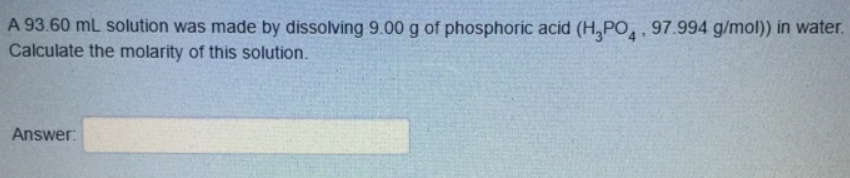# Problem: A 93.60 mL solution was made by dissolving 9.00 g of phosphoric acid (H  3PO4, 97.994 g/mol)) in water. Calculate the molarity of this solution. Answer:

###### FREE Expert Solution
90% (6 ratings)###### Problem Details

A 93.60 mL solution was made by dissolving 9.00 g of phosphoric acid (H  3PO4, 97.994 g/mol)) in water.

Calculate the molarity of this solution. Answer: